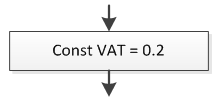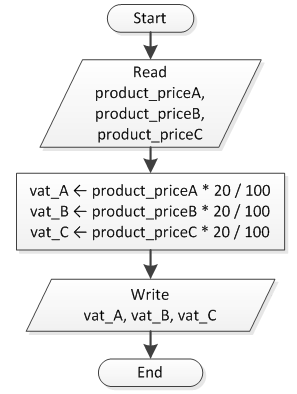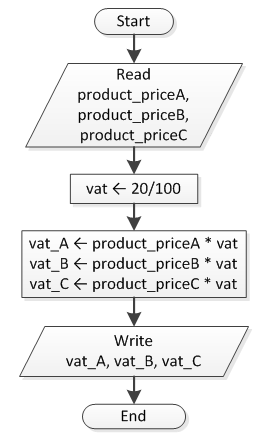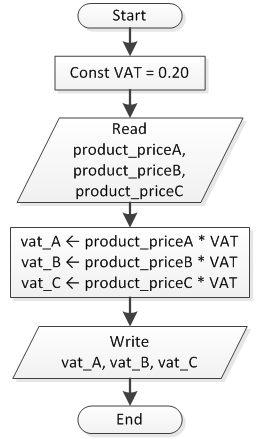Sometimes you may need to use a value that cannot change while the program is running. Such a value is called a “constant.” In simple terms, you could say that a constant is a locked variable. This means that when a program begins to run and a value is assigned to the constant, nothing can change the value of the constant while the program is running. For example, in a financial program an interest rate can be declared as a constant.

A descriptive name for a constant can also improve the readability of your program and help you avoid errors. For example, let’s say that you are using the value 3.14159265 (but not as a constant) at many points throughout your program. If you make an error when typing the number, this will produce the wrong results. But, if this value is given a name, any typographical error in the name is detected by PHP, and you are notified with an error message.

In a flowchart, you can represent the action of setting a constant equal to a value with the equals sign, ( = ).Notice: You can use the reserved word` Const `to distinguish a constant from a variable.

Consider an algorithm that lets the user enter the prices of three different products and then calculates and displays the 20% Value Added Tax (known as VAT) for each product. The flowchart in Figure 1 shows this process when no constant is used.Figure 1 Calculating the 20% VAT for three products without the use of a constant

Even though this algorithm is absolutely correct, the problem is that the author used the 20% VAT (20/100) three times. If this were an actual computer program, the CPU would be forced to calculate the result of the division (20/100) three individual times.

Notice: Generally speaking, division and multiplication are time consuming operations that must be avoided when possible.

A much better solution would be to use a variable, as shown in Figure 2. This reduces the number of division and multiplication operations and also decreases the potential for typographical errors.Figure 2 Calculating the 20% VAT for three products using a variable, vat

This time the division (20/100) is calculated only once, and then its result is used to calculate the VAT of each product.

But even now, the algorithm (which might later become a computer program) isn’t perfect;` vat` is a variable and any programmer could accidentally change its value.

The ideal solution would be to change the variable` vat` to a constant` VAT`, as shown in Figure 3.Figure 3 Calculating the 20% VAT for three products using a constant, VAT

Notice: Please note that when a constant is declared, the equals symbol ( = ) is used instead of the left arrow.

This last solution is the best choice for many reasons.

• No one, including the programmer, can change the value of` VAT` just by accidentally writing a statement such as` VAT ← 60` in any position of the program.
• The potential for typographical errors is minimized.
• The number of multiplication and division operations is kept as low as possible.
• If one day the finance minister decides to increase the Value Added Tax from 20% to 22%, the programmer needs to change just one line of code!